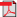# Search Results

1. E. Nyberg, Calculation of Blocking Probabilities and Dimensioning of Multicast Networks, Master's Thesis, Networking Laboratory, Helsinki University of Technology, 1999(bib)
Abstract: This thesis is a study on mathematical models for calculating blocking probabilities and dimensioning of multicast networks. Multicasting is a bandwidth saving transmission technique designed for group applications such as video conferencing. The transmission reaches many different end-users without a separate connection required for each user. Due to this characteristic, conventional blocking probability analysis of loss networks has to be modified. The study derives a new algorithm for calculating blocking probabilities in dynamic multicast networks. The exact algorithm is based on the well-known algorithm for calculating blocking probabilities in hierarchical access networks. The algorithm is modified by introducing a new convolution method, the OR-convolution. The algorithm is applied to an existing infinite user population multicast model, for which until now only a single link solution has been given. The study derives a new finite user population model, which is in accordance with the infinite user population model, and applies the algorithm to this new network model. The algorithm is further extended by taking into account the effect of non-multicast traffic, background traffic, in the network. The dimensioning of the network is studied using the finite population model. An optimum capacity allocation is found for an example network using Moe's principle. Due to the complexity of the exact algorithm, the use of an approximate method, the Reduced Load Approximation (RLA), is studied. The work assumes that the reader has a basic understanding of Probability theory and Stochastic processes. The theory behind Stochastic processes and network protocols are however briefly introduced.